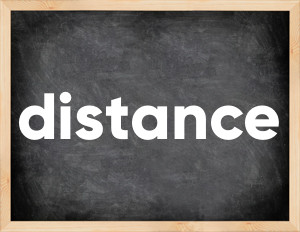# Distance past tenseThe English verb 'distance' is pronounced as [ˈdɪstəns].
Related to: regular verbs.
3 forms of verb distance: Infinitive (distance), Past Simple - (distanced), Past Participle - (distanced).

## Here are the past tense forms of the verb distance

👉 Forms of verb distance in future and past simple and past participle.
❓ What is the past tense of distance.

## Distance: Past, Present, and Participle Forms

Base Form Past Simple Past Participle
distance [ˈdɪstəns]

distanced [ˈdɪstənst]

distanced [ˈdɪstənst]

## What are the 2nd and 3rd forms of the verb distance?

🎓 What are the past simple, future simple, present perfect, past perfect, and future perfect forms of the base form (infinitive) 'distance'?

### Learn the three forms of the English verb 'distance'

• the first form (V1) is 'distance' used in present simple and future simple tenses.
• the second form (V2) is 'distanced' used in past simple tense.
• the third form (V3) is 'distanced' used in present perfect and past perfect tenses.

## What are the past tense and past participle of distance?

The past tense and past participle of distance are: distance in past simple is distanced, and past participle is distanced.

### What is the past tense of distance?

The past tense of the verb "distance" is "distanced", and the past participle is "distanced".

### Verb Tenses

Past simple — distance in past simple distanced (V2).
Future simple — distance in future simple is distance (will + V1).
Present Perfect — distance in present perfect tense is distanced (have/has + V3).
Past Perfect — distance in past perfect tense is distanced (had + V3).

### distance regular or irregular verb?

👉 Is 'distance' a regular or irregular verb? The verb 'distance' is regular verb.

## Examples of Verb distance in Sentences

•   It distanced her from the narrow group of key partners. (Past Simple)
•   They ordered all fronts to distance themselves from conflict sites. (Past Simple)
•   He has made certain decisions that have distanced him from me. (Present Perfect)
•   They've actually distanced us from our most important relationship, which is that of us and nature. (Present Perfect)
•   This further distanced her from political power and jeopardized the future of her own leaders. (Past Simple)
•   And you said yourself that she has distanced from you. (Past Perfect)
•   I distanced myself, said I needed a break. (Past Simple)
•   The government and the opposition should clearly distance themselves from such tactics. (Present Simple)
•   The VP is looking to distance himself from this trade deal. (Present Continuous)
•   We can choose to distance ourselves from them. (Present Simple)

Along with distance, words are popular doze and define.

Verbs by letter: , , , , , , , , , , , , , , , , , , , , , , , , .